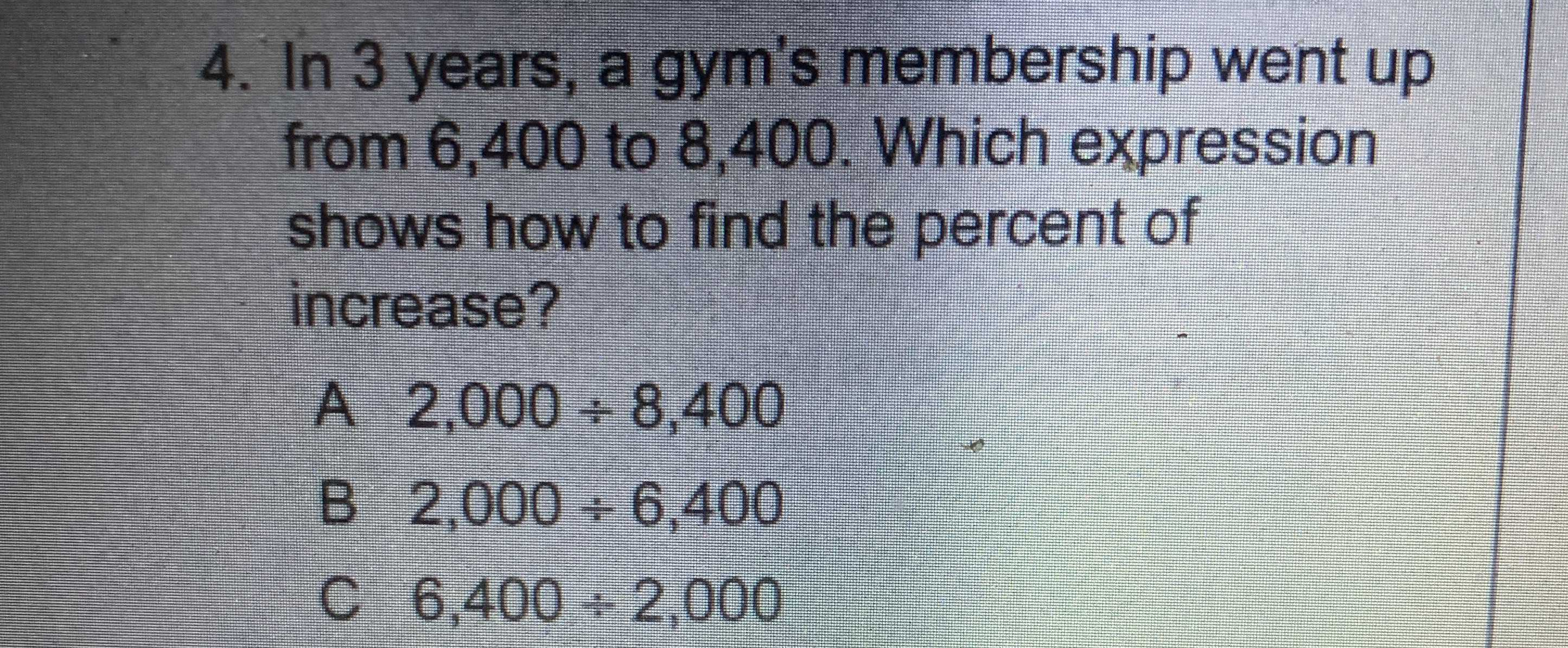### ¿Todavía tienes preguntas de matemáticas?

Pregunte a nuestros tutores expertos
Algebra
Pregunta4. In $$3$$ years, a gym's membership went up from $$6,400$$ to $$8,400$$ . Which expression shows how to find the percent of increase?

$$2,000 \div 8,400$$

$$2,000 \div 6,400$$

$$6,400 \div 2,000$$